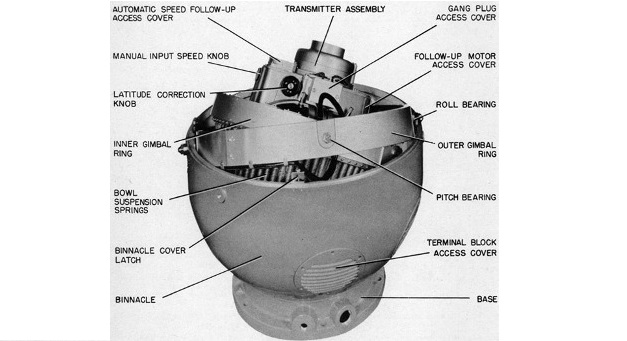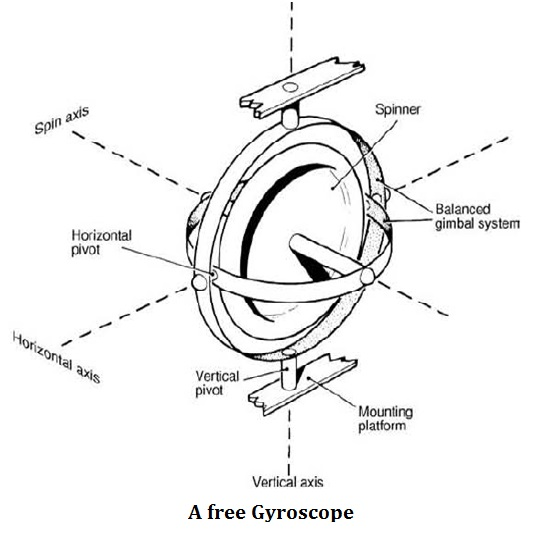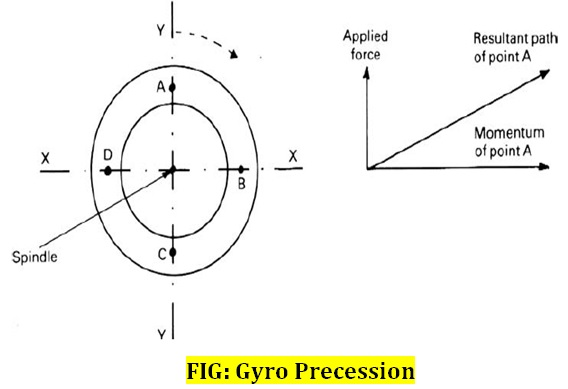Miscelleneous

# What is Gyro Compass ?Written by

A marine gyro compass assembly is a modern gyroscope designed to automatically find geographical directions. Although gyroscope is one important component of a gyro compass, these are not the same devices; a gyro compass is built to use the effect of gyroscopic precession, which is a distinctive aspect of the general gyroscopic effect. Gyroscope consists of a perfectly balanced wheel arranged to spin symmetrically at high speed about an axis or axle. The wheel, or rotor, spins about its own axis and, by suspending the mass in a precisely designed gimbals assembly, the unit is free to move in two planes each at right angles to the plane of spin. There are therefore three axes in which the gyroscope is free to move.

• The spin axis.
• The horizontal axis.
• The vertical axis.###### Basic Operation of free Gyroscope

A free gyroscope possesses certain inherent properties, one of which is inertia, a phenomenon that can be directly related to one of the basic laws of motion documented by Newton. Newton’s first law of motion states that ‘a body will remain in its state of rest or uniform motion in a straight line unless a force is applied to change that state’. Therefore a spinning mass will remain in its plane of rotation unless acted upon by an external force. Consequently the spinning mass offers opposition to an external force. This is called ‘gyroscopic inertia’

A gyroscope rotor maintains the direction of its plane of rotation unless an external force of sufficient amplitude to overcome inertia is applied to alter that direction.

###### What are factors on which gyroscopic inertia depend?

Gyroscopic inertia depends upon the momentum of the spinning rotor. The momentum of such a rotor depends upon three main factors:

• The total mass, M of the rotor (for all particles).
• The radius r summed as the constant K (for all the particles) where K is the radius of gyration.
• The angular velocity.
###### Precession

Precession is the term used to describe the movement of the axle of a gyroscope under the influence of an external force. If a force is applied to the rotor by moving one end of its axle, the gyroscope will be displaced at an angle of 90° from the applied force